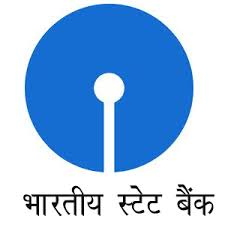May 15, 2016

# SBI Junior Associate Quantitative Aptitude Practice Paper## State Bank of India Junior Associate Clerk Grade Practice Paper

1. 75% of a number is equal to three-seventh of another number. What is the ratio between the first number and the second number respectively?
A. 4 : 7
B. 7 : 4
C. 12 : 7
D. 7 : 12
E. None of these

2. A 275 meter long train crosses a platform of equal length in 33 seconds. What is the speed of the train in kmph?
A. 66
B. 60
C. 64
D. 72
E. None of these

3. What is compound interest accrued on an amount of Rs. 45,000/- in two years @ 9 percent per annum?
A. Rs. 8,600/-
B. Rs. 8,565.4O/-
C. Rs. 8,464.50/-
D. Rs. 8,540/-
E. None of these

4. Cost of 18 shirts and 45 trousers is Rs. 68,400/-. What is the cost of 10 shirts and 25 trousers?
A. Rs. 38,000/-
B. Rs. 36,000/-
C. Rs. 34,200/-
D. Rs. 36,200/-
E. None of these

5. If the fractions 9/11, 7/9, 5/6, 4/5 and 11/13 are arranged in ascending order, which one will be the fourth?
A. 9/11
B. 7/9
C. 5/6
D. 4/5
E. 11/13

6. Simple interest accrued on an amount in 8 years @ 12 per cent per annum is Rs. 5,520/-. What is the principal amount?
A. Rs. 5,750/-
B. Rs. 8,500/-
C. Rs. 5,650/-
D. Rs. 8,250/-
E. None of these

## SBI Junior Assoicate Examination Questions Answers

Latest Sample Paper SBI Junior Associate Examination
Model Question Paper State Bank of India Junior Assistant
SBI Junior Assistant Computer Aptitude Question Paper
SBI Junior Associate English Language Model Question Paper
SBI Junior Associate Preliminary English Sample Question 2016
SBI Junior Associate Preliminary Aptitude Sample Question 2016
SBI Junior Associate Preliminary Reasoning Sample Question 2016
SBI Junior Associate Reasoning Question Paper
SBI Junior Associate Quantitative Aptitude Question Paper
SBI Junior Agricultural Associate Model Question Paper
SBI Junior Agricultural Associates Preliminary Sample Question
SBI Junior Agricultural Associates Reasoning Question

7. Find the average of the following set of number:
148, 88, 184, 166, 96, 122
A. 146
B. 142
C. 136
D. 132
E. None of these

8. Shrikant and Vividh started a business investing amounts of Rs. 1,85,000/- and Rs. 2,25,000/- respectively. If Vividh’s share in the profit earned by them is Rs. 9,000/-, what is the total profit earned by them together?
A. Rs. 17,400/-
B. Rs. l6,400/-
C. Rs. 16,800/-
D. Rs. l7,800/-
E. None of these

9. Present ages of father and the son are in the ratio of 6 : 1 respectively. Four years hence the ratio of their ages will become 4 : 1 respectively. What is the son’s present age?
A. 10 years
B. 6 years
C. 4 years
D. 8 years
E. None of these

10. A DVD player was purchased for Rs; 4,860/-. At what price should it be sold so that 25% profit is earned?
A. Rs. 6,225/-
B. Rs. 6,275/-
C. Rs. 6,075/-
D. Rs. 6,025/-
E. None of these

11. 65% of a number is more than its two-fifth by 140. What is 30% of that number?
A. 186
B. 168
C. 164
D. 182
E. None of these

12. Number obtained by interchanging the digits of a two digit number is more than the original number by 27 and the sum of the digits is 13. What is the original number?
A. 58
B. 67
C. 76
D. 85
E. None of these

13. 22 Men can complete a job in 16 days. In how many days will 32 men complete that job?
A. 14
B. 12
C. 16
D. 9
E. None of these

14. Mr. Davar spends 38% of his monthly income on food, 25% on children’s education and 12% on transport and the remaining amount of Rs. 5,800/- he saves. What is Mr. Davar’s monthly income?
A. Rs. 23,200/-
B. Rs. 24,200/-
C. Rs. 23,800/-
D. Rs. 24,400/-
E. None of these

15. A, B, C, D and E are five consecutive odd numbers. Average of A and C is 59. What is the smallest number?
A. 65
B. 63
C. 61
D. 57
E. None of these

Qs. 16 to 20: Study the following table carefully to answer these questions

Number of students appeared in SSC examination from five schools over the years
 School → A B C D E Year ↓ 2004 650 760 820 800 780 2005 700 740 860 780 740 2006 800 820 940 750 730 2007 750 880 920 840 790 2008 850 840 900 860 770

16. Number of students appeared from school E in 2004 is approximately what percent of the total number of students appeared from all the schools together in that year?
A. 12
B. 28
C. 15
D. 30
E. 20

17. What is the average number of students appeared from school B for all the years?
A. 828
B. 808
C. 804
D. 812
E. None of these

18. Number of students appeared in 2006 from school A is what percent of the total number of students appeared from school A for all the years together?
A. 23(2/3)
B. 22(1/3)
C. 22(2/3)
D. 21(1/3)
E. None of these

19. What is the ratio between the total numbers of students appeared in 2004 and 2005 together from schools C and D respectively?
A. 84:79
B. 79:84
C. 48:79
D. 79:48
E. None of these

20. What is the average number of students appeared from all the schools in 2007?
A. 842
B. 856
C. 836
D. 830
E. None of these

 Questions Answers 1 A 2 B 3 C 4 A 5 C 6 A 7 E 8 B 9 B 10 C 11 D 12 B 13 E 14 B 15 C 16 D 17 E 18 A 19 D 20 D